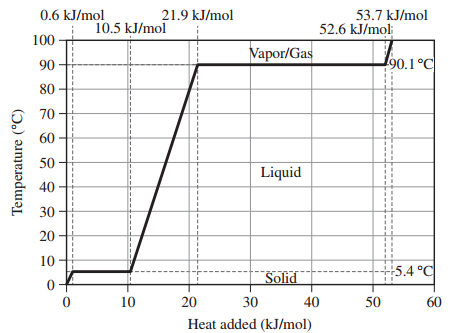# Problem: Assume that the values given here for 1 mole of benzene are constant over the relevant temperature ranges.Melting point5.4 oCBoiling point90.1 oCΔHfus9.9 kJ/molΔHvap30.7 kJ/molCs,solid118 J/mol • KCs,liquid135 J/mol • KCs,gas104 J/mol • KWhat is the qgas value for 1 mole of benzene?

###### FREE Expert Solution
• The heat involved (Qgas) upon heating gaseous benzene from 90°C to 100°C can be calculated as:

95% (476 ratings)###### Problem Details

Assume that the values given here for 1 mole of benzene are constant over the relevant temperature ranges.

 Melting point 5.4 oC Boiling point 90.1 oC ΔHfus 9.9 kJ/mol ΔHvap 30.7 kJ/mol Cs,solid 118 J/mol • K Cs,liquid 135 J/mol • K Cs,gas 104 J/mol • KWhat is the qgas value for 1 mole of benzene?